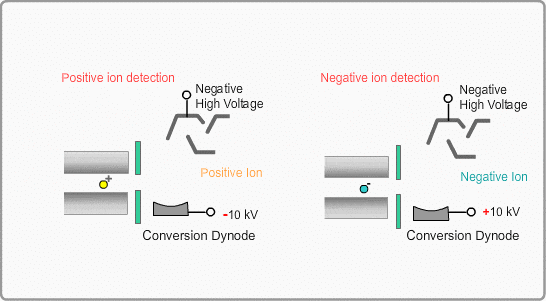# Detector

## Overview of Detector System

The ions separated by the quadrupole mass filter are detected by the detector, which converts ions to electrons (electric current). Then the current is processed by an electric circuit. The most popular detector is an electron multiplier that multiplies the number of electrons emitted from the surface by impingement of ions to increase the signal intensity.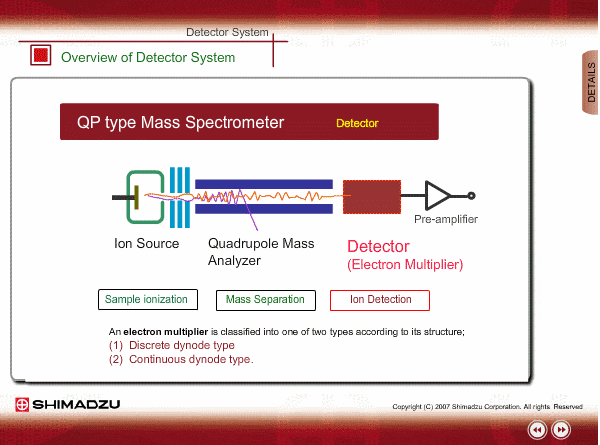## Structure of Electron Multiplier (Discrete-Dynode Type)

An electron multiplier consists of many dynodes. Negative voltage is applied to each one by dividing the high voltage applied to the first dynode with resisters. A few secondary electrons are emitted from the first dynode by ion impingement. An electron multiplier is put in an off-axis position to reduce noise signals by direct hit of neutral particles.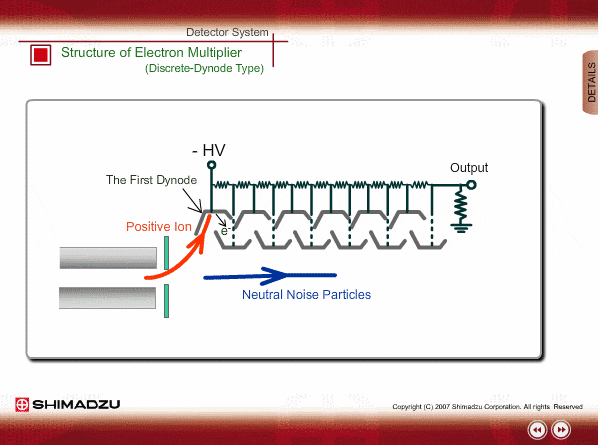## Electron Multiplication

When the average number of secondary electrons emitted from each dynode, which depends on the potential difference between dynodes, is more than one, electron multiplication occurs in the electron multiplier. The typical discrete multiplier has between 10 and 25 dynodes and is operated at a gain of between 104 and 108.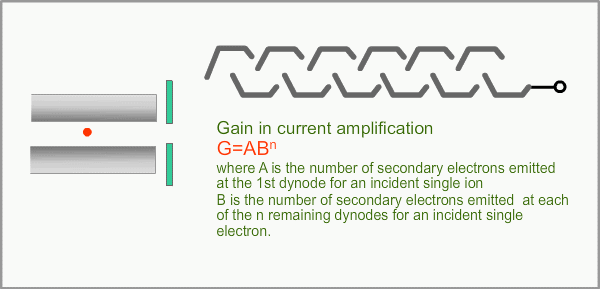## Gain of Electron Multiplier

The gain control is performed by changing the high voltage applied to the first dynode. The applied voltages to other dynodes are automatically determined by the resisters mounted in the multiplier. The typical gain curve of a new multiplier is shown below. In general, the gain gradually decreases after operating the multiplier for a long time. It is better to avoid operating at unnecessary and excessive high gain to extend the multiplier’s life.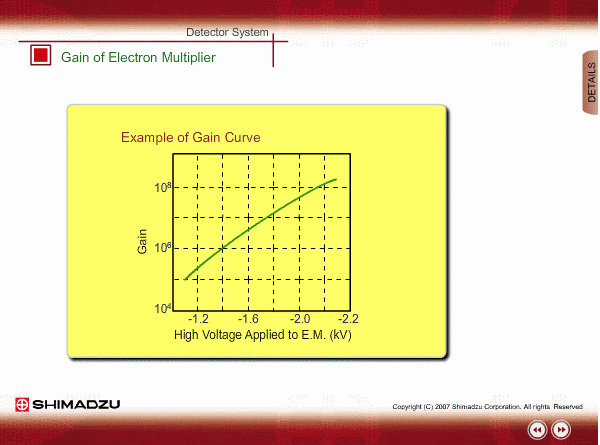## Structure of Electron Multiplier (Continuous-Dynode Type)

The continuous-dynode type sometimes is in the shape of a horn. This type of multiplier is made by extruding lead-silicate glass. The multiplication principle is similar to the discrete-dynode type, but electrons are emitted from the continuous inner surface of the horn, instead of from a discrete metal dynode. There are no discrete resistors, because the film of the inner surface is a spatial resistance itself.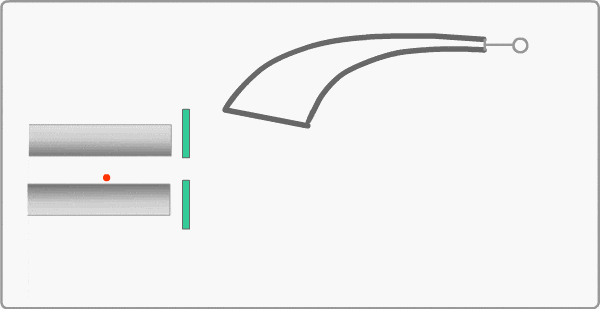## Conversion Dynode

A conversion dynode is sometimes put in front of the electron multiplier. This dynode, on which a high voltage of 5 to 20 kV with reverse polarity to the ion is applied, makes it possible to detect negative ions and increase the signal intensity of ions, particularly in the high mass region.